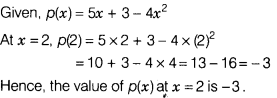# Find the value of polynomial p(x) = 5x + 3 - 4x2 at x = 2

Find the value of polynomial p(x) = 5x + 3 - 4x2 at x = 2.